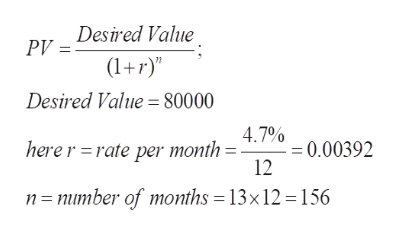Use the present value formula to determne the amt to be invested now, or the present value neededThe desired accumulated amt is 80,000 after 13 years investedin an account with 4.7% interest compounded monthly./The amt to be invested now, or the present value needed is___________​(Round to the nearest cent as​ needed.)

Question

Use the present value formula to determne the amt to be invested now, or the present value needed

The desired accumulated amt is 80,000 after 13 years investedin an account with 4.7% interest compounded monthly./

The amt to be invested now, or the present value needed is___________

​(Round to the nearest cent as​ needed.)
Step 1

To calculate the present value (PV) (the amount to be invested now) which yields the desired return under the given contions

Step 2

Formula for the prese...help_outlineImage TranscriptionclosePV Desired Value (1)" Desired Value = 80000 4.7% = 0.00392 12 here r rate per month n = mimber of months = 13x 12 = 156 fullscreen

Want to see the full answer?

See Solution

Want to see this answer and more?

Our solutions are written by experts, many with advanced degrees, and available 24/7

See Solution
Tagged in

Math